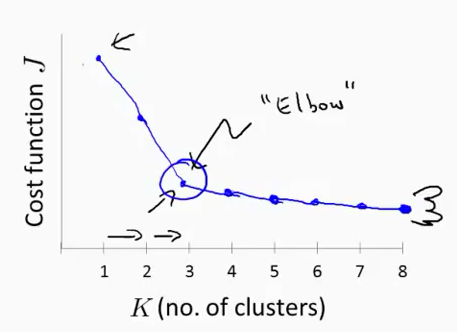前言

K-均值是一種無監督的聚類演算法。首先我們要知道什麼是無監督，無監督就是說在資料集中，資料是沒有標籤的。在有監督的資料集中，資料的形式可能是這樣：{(x(1),y(1)),(x(2),y(2)),...,(x(m),y(m))}。而在無監督的資料集中，資料的形式是：{x(1),x(2),...,x(m)}。所謂的標籤，就是有沒有y。

K-均值演算法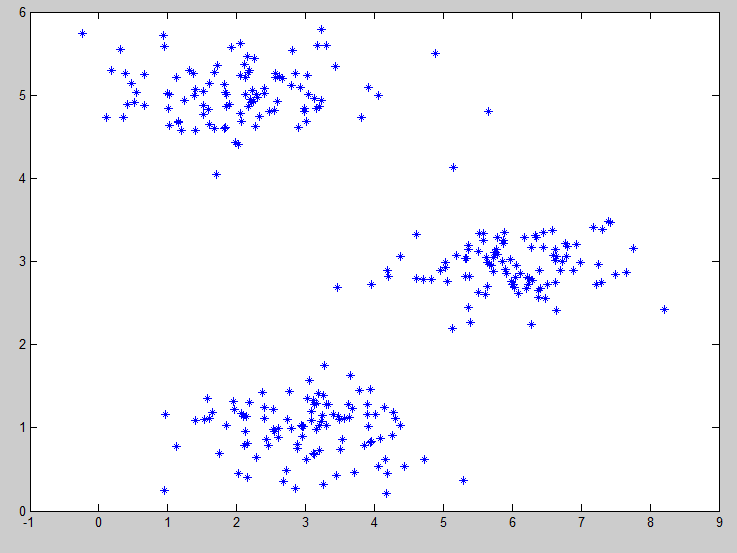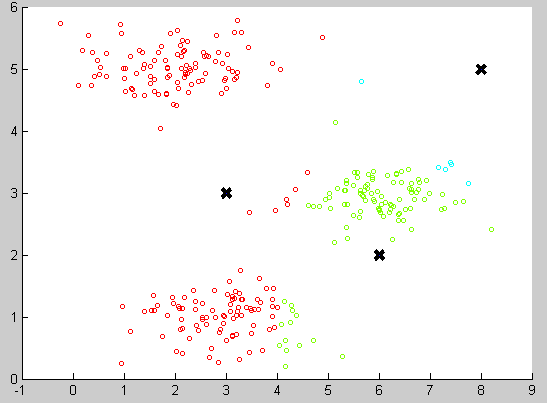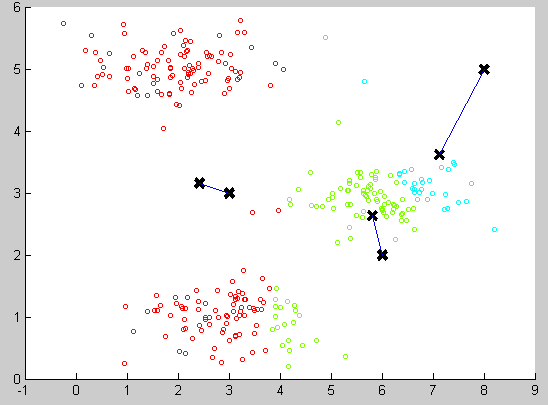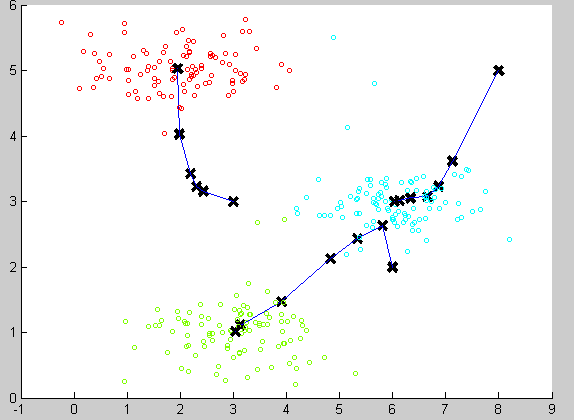演算法步驟與虛擬碼

K-均值的虛擬碼如下：
μ1,μ2,...,μK來表示聚類中心，用c(1),c(2),...,c(m)來儲存於第i個訓練資料最近的聚類中心的索引(即從1到K的某一個數)，

Repeat{
for i=1 to m
c(i) := index(from 1 to K )of cluster centroid closest to x(i)

for k = 1 to K
μk := average(mean) of points assigned to cluster k
}

優化目標（代價函式）

K-均值最小化問題，是要最小化所有的資料點與其關聯的聚類中心之間的距離之和。因此，K-均值的代價函式：

J(c(1),c(2),...,c(m),μ1,μ2,...,μK)=1mi=1mx(i)μc(i)2

初始化問題

1. 首先，應該選擇K < m，也就是聚類中心的個數要小於所有訓練集例項的數量。
2. 隨機選擇K個訓練樣本，然後令K個聚類中心分別與這K個訓練樣本相等。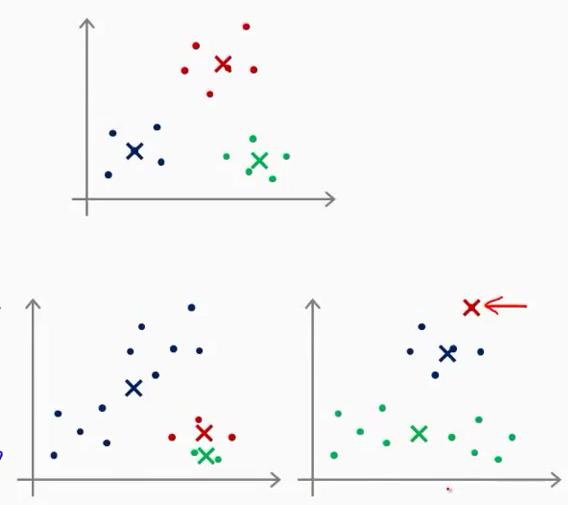K的選擇

肘部法則